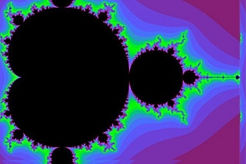top of page## Velocity as basic parameter

Fractal heuristic ideas on emergenceEach generation passes on to the following generation four essential qualities: its constants, its impulses, its transformations, and what emerges in it (emergence).

The constants should be scalars, the impulses must be expressed as usual in the natural sciences according to an equation I = m * v, where m is the mass and v the velocity. Transformations can be seen in the kinetic energy (const) m * v2, which is found in extreme form in the Einstein relation E = mc2, which accounts for the important conversion of mass into energy, e.g. in reactors and nuclear weapons.

Thus we have a constant, a linear and a quadratic term of a beginning series expansion as a function of a quantity x, which is obviously the velocity. However, according to the considerations outlined on this website, our world is to be described as four-dimensional, so that a cubic term would have to be added as well, ie of the form m * v3, which does not mean anything to us at the moment, but should contain emergence. Overall, we can write:

m0 + m1 * xn + m2 * xn2 + m3 * xn3 ==> xn + 1

which would mean that a new velocity can be derived in each new generation, which would be a function of the velocity of the previous generation.

Speculation is frowned upon in the natural sciences, but has its legitimacy as a heuristic agent. Since, at least for the time being, no proof can be given for these considerations, consistency should at least be required. In any case, the above formula makes it understandable that the velocity (for example, of the growth of the universe we know) always goes on to increase.

The exciting question, however, would be whether there may be a third-power term of velocity which in any way should describe emergence, e.g. in a supernova with possible superfluidity or in pair-formation (e.g., electron and positron). All in all, however, fractal ideas should also be attributed the same importance as to logically derived findings. Beyond a certain complexity these in any case go beyond strict logic and are in agreement to notions of "fuzzy" logic. Heuristic approaches to so-called "fractomatics" can also be found on this website.